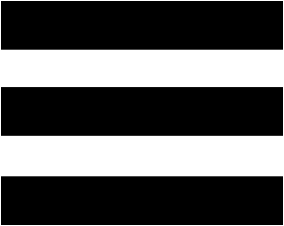RM. Palaniappan @ 9047020818 / 04565-227818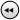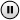WELCOME TO KARAIKUDI PROPERTIES! | We welcome your suggestions and feedback to improve the quality of information we provide. Now you have a trusted platform to avail our services.

### Avena Apartments : Block Details

Block A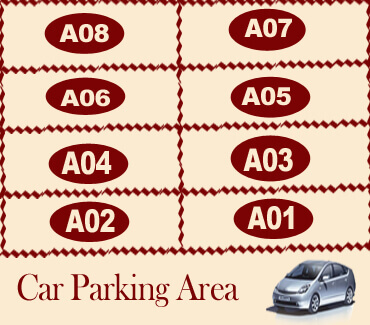Ground Floor

A01 = 974.51

A02 = 940.01

First Floor

A03 = 1144.87

A04 = 1020.00

Second Floor

A05 = 1144.87

A06 = 1020.00

Third Floor

A07 = 1144.87

A08 = 1020.00

Block B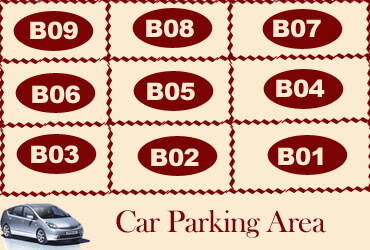Ground Floor

Car Parking Area

First Floor

B01 = 1016.46

B02 = 607.08

B03 = 584.56

Second Floor

B04 = 1016.46

B05 = 607.08

B06 = 584.56

Third Floor

B07 = 1016.46

B08 = 607.08

B09 = 584.56

Block C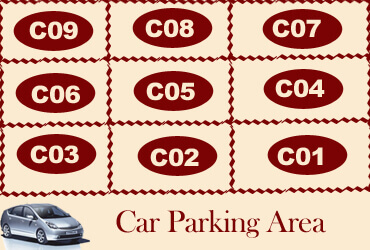Ground Floor

Car Parking Area

First Floor

C01 = 1016.46

C02 = 607.08

C03 = 584.56

Second Floor

C04 = 1016.46

C05 = 607.08

C06 = 584.56

Third Floor

C07 = 1016.46

C08 = 607.08

C09 = 584.56